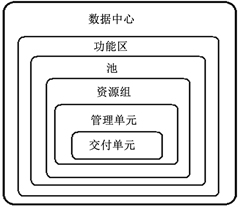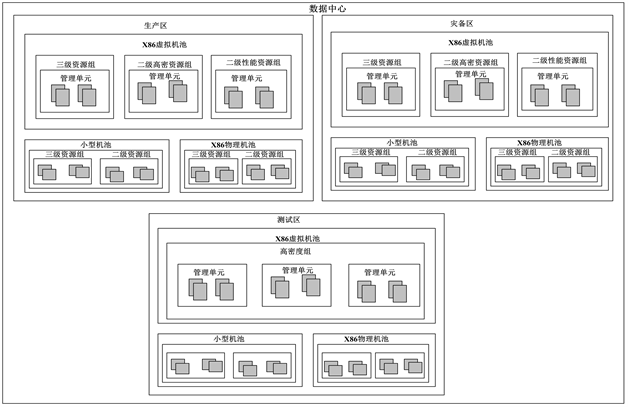# 电力云资源池投资成本监控算法研究Study on the Investment Cost Monitoring Algorithm of Electric Cloud’s Resource Pool

DOI: 10.12677/SG.2018.85047, PDF, HTML, XML, 下载: 440  浏览: 561  科研立项经费支持

Abstract: This paper studied the base schema of electric cloud’s resource pool and made clearly the key fac-tors of resource pool. Base on this, this paper analyzed the investment const of resource pool and built the investment cost model. Compared with the traditional information construction, the in-vestment cost monitoring algorithm was also built. The investment cost model can compute the investment cost of different scope resource pool, the investment cost monitoring algorithm can monitor the investment cost, and the algorithm can get the condition of getting positive economic benefit and how much it can gain. Enterprises can monitor and analyze the investment cost and the gain, and can get the direction of the investment deviation and node. At the same time, enterprises would take effective and remedial measures to revise.

1. 引言

2. 电力云资源池的基础架构Figure 1. Six-layer physical model of resource poolFigure 2. The basal architecture of one data center’s resource pool

3. 电力云资源池投资成本监控算法

3.1. 电力云资源池投资成本监控算法

$Y\left(x\right)=T\left(x\right)-Z\left(x\right)$ (1)

3.2. 成本分析

1) 资源池投资成本分析

${C}_{h}\left(x\right)={c}_{\mathrm{min}}\left(x\right)×N$ (2)

${C}_{R}\left(x\right)=\text{N}×\varphi ×100,000$ (3)Table 1. Composition cost of the typical mini-delivery unit. Unit: ten thousand Yuan

${C}_{i}\left(x\right)=\beta ×N$ (4)

$Z\left(x\right)={C}_{h}\left(x\right)+{C}_{r}\left(x\right)+{C}_{i}\left(x\right)$ (5)

2) 同等能力传统架构建设的成本

${C}_{h}^{t}\left(x\right)=3×N×{c}_{s}$ (6)

${C}_{r}^{t}\left(x\right)+{C}_{i}^{t}\left(x\right)={C}_{r}\left(x\right)+{C}_{i}\left(x\right)$ (7)

3.3. 算法的实践与分析

$Y\left(x\right)=3×N×{c}_{s}-{c}_{\mathrm{min}}×N$ (8)Table 2. Financial rewards of different scale resource pool. Unit: ten thousand YuanFigure 3. Investment cost and rewards of different construction scale

4. 结论及展望

NOTES

*通讯作者。

  朱征, 顾中坚, 吴金龙, 等. 云计算在电力系统数据灾备业务中的应用研究[J]. 电网技术, 2012, 36(9): 43-50.  王德文. 基于云计算的电力数据中心基础架构及其关健技术[J]. 电力系统自动化, 2012, 36(11): 67-71.  沐连顺, 崔立忠, 安宁. 电力系统云计算中心的研究与实践[J]. 电网技术, 2011, 35(6): 171-175.  赵俊华, 文福栓, 薛萬胜, 等. 云计算: 构建未来电力系统的核心计算平台[J]. 电力系统自动化, 2010, 34(15): 1-8.  王健伟. 一种全新的IT建设架构—统一资源池[J]. 数据通信, 2011(5): 10-12.  百云川, 钱敏谨. 利用虚拟化技术构建资源池是实现云计算的第一步[J]. 中国制造业信息化, 2010(12): 34.  张科, 董亮, 邹澄澄. 利用云计算技术建立电力信息系统硬件资源池[J]. 湖北电力, 2014, 38(6): 1-3.  姚岳. 运营商业务平台云计算资源池建设方案探讨[J]. 电信技术, 2015(4): 39-45.  董亮, 詹伟, 袁慧, 等. 湖北省电力公司云硬件资源池系统建设实践[J]. 信息与电脑, 2015(15): 87-90.  李小康. IaaS资源池管理平台的设计与实现[D]: [硕士学位论文]. 北京: 北京邮电大学, 2013.  洪枯荣. 企业云计算应用的成本效用分析与决策[D]: [硕士学位论文]. 哈尔宾:哈尔宾商业大学, 2015.  刘营, 周丽媛, 陇小渝. 企业接入云计算的成本分析[J]. 电子信, 2011(10): 295.  刘森. 企业云计算信息化的投资回报率分析[J]. 中国科技论坛, 2014(6): 83-87.